### 6. The kinematic Sunyaev-Zel'dovich effect

Although early work on the Sunyaev-Zel'dovich effects concentrated on the thermal effect, a second effect must also occur when the thermal (or non-thermal) Sunyaev-Zel'dovich effect is present. This is the velocity (or kinematic) Sunyaev-Zel'dovich effect, which arises if the scattering medium causing the thermal (or non-thermal) Sunyaev-Zel'dovich effect is moving relative to the Hubble flow. In the reference frame of the scattering gas the microwave background radiation appears anisotropic, and the effect of the inverse-Compton scattering is to re-isotropize the radiation slightly. Back in the rest frame of the observer the radiation field is no longer isotropic, but shows a structure towards the scattering atmosphere with amplitude proportional toe vz / c, where vz is the component of peculiar velocity of the scattering atmosphere along the line of sight (Sunyaev & Zel'dovich 1972; Rephaeli & Lahav 1991).

The most interesting aspect of the kinematic effect is that it provides a method for measuring one component of the peculiar velocity of an object at large distance, provided that the velocity and thermal effects can be separated, as they can using their different spectral properties. Since there is evidence for large-scale motions of clusters of galaxies in the local Universe, both from COBE (Fixsen et al. 1996) and from direct observations of galaxies (e.g., Dressler et al. 1987; Lynden-Bell et al. 1988), and these motions place strong constraints on the dynamics of structure formation (see the review of Davis et al. 1992), other examples of large-scale flows would be of considerable interest. This is particularly true if those flows can be measured over a range of redshifts, so that the development of the peculiar velocity field can be studied. At present this is beyond the capability of the measurements (Sec. 8), but rapid progress is being made in this area.

Even larger velocities are possible for scattering gas: radio source lobes may be moving at speeds that approach the speed of light, and expanding at hundreds or thousands of km s-1. Although this will boost the kinematic effect, the optical depths of these lobes are probably too small to make the effect observable at present (Sec. 5; Molnar 1998).

Although Sunyaev & Zel'dovich (1972) quoted the result that the radiation temperature decrease in the kinematic effect is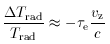(79)

and the spectrum of the kinematic effect has often been quoted (e.g., by Rephaeli 1995b), the first published derivation of the size and spectrum of the kinematic effect was given by Phillips (1995). The version of the derivation given here is similar to Phillips' argument, but uses different conventions and the radiative transfer equation (19) rather than the Boltzmann equation.

For the sake of simplicity, it is assumed that the kinematic and the thermal effects are both small, and that only single scatterings are important. Then the thermal effect, which depends on random motions of the scattering electrons, and the kinematic effect, which depends on their systematic motion, will decouple and we can derive the kinematic effect by taking the electrons to be at rest in the frame of the scattering medium. This approximation will ignore quantities which involve cross-products [(kB Te) / (me c2)] (vz / c) relative to terms of order [(kB Te) / (me c2)] and (vz / c). Since the peculiar velocities and electron temperatures are small for the thermal Sunyaev-Zel'dovich effect, this will not be a significant limitation on the result for clusters of galaxies. However, this approximation is not valid for the non-thermal effect, where electron energies >> me c2 are likely, and an alternative analysis is necessary (Molnar 1998; Nozawa et al. 1998).

In the rest frame of the CMBR, the spectrum of the radiation follows (1), and the occupation number has the form (15). The occupation number in modein a frame moving at speed vz along the z axis away from the observer is then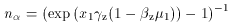(80)

where x1 = (h1) / (kB T1) is the dimensionless frequency of photons in the frame of the scattering medium, the radiation temperature of the CMBR as seen by an observer at rest in the Hubble flow near the scattering gas is T1 = Trad (1 + zH), zH is the Hubble flow redshift, andz = vz / c measures the peculiar velocity.z is the corresponding Lorentz factor. µ1 = cos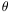1 is the direction cosine of photons arriving at a scattering electron, relative to the z axis and measured in the frame of the moving scattering medium (Fig. 12). The relativistic transformation of frequency relates1 toin the frame at rest relative to the CMBR by1 =z ( 1 +z), since the observer at rest sees the scattered photons along the z axis, where µ = cos= 1.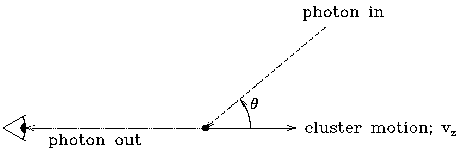Figure 12. The geometry for the discussion of the kinematic Sunyaev-Zel'dovich effect, as seen in the frame of an observer at rest in the Hubble flow.

We may apply the Boltzmann equation (e.g., as in Peebles 1993, Sec. 24 and Phillips 1995), or the radiative transfer equation (19), to derive an equation for the scattered radiation intensity. Using (19), and the form (24) for the scattering redistribution function, the specific intensity is given by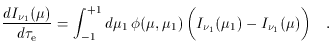(81)

The optical depth,e, enters from (19) ase =,sca dz. For small optical depth, so that photons are scattered only once, this can be simplified to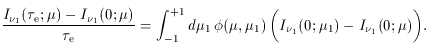(82)

where the optical depth is now inserted as an explicit argument of I. For µ = 1, the scattering redistribution function takes a particularly simple form, and we can write the fractional change in the specific intensity in the frame of the scattering gas as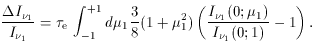(83)

The expression on the left-hand side of this equation is a relativistic invariant: the same fractional intensity change would seen by an observer in the rest frame of the CMBR at frequency, whereis related to1 by a Lorentz transform. Furthermore, this is also the fractional intensity change seen by a distant observer, for whom the scattering medium lies at redshift zH, after allowance is made for the redshifting of frequency and radiation temperature. Using the expression for nin (80), and working in terms of the frequency seen at redshift zero,, (83) becomes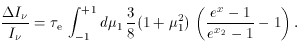(84)

where x2 = xz2 (1 +z) ( 1 -z µ1) and x = (h) / (kB Trad) as usual.

For smallz, the integral can be expanded in powers ofz, and the symmetry of the integrand ensures that only terms in the expansion which are even powers of µ1 will appear in the result. This enables the integral to be performed easily, giving the result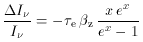(85)

so that the changes in specific intensity and brightness temperature are given by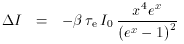(86)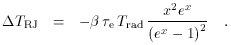(87)

This spectral form corresponds to a simple decrease in the radiation temperature (79), as stated by Sunyaev & Zel'dovich (1972).

For the cluster CL 0016+16 discussed in Sec. 4, the X-ray data imply a central scattering optical depthe0 = 0.01 h100-1/2. At low frequency the brightness temperature change through the cluster center caused by the kinematic effect is -e0 (vz / c) Trad = - 0.1 (vz / 1000 km s-1) h100-1/2 mK, significantly less than the central thermal Sunyaev-Zel'dovich effect of -0.82 h100-1/2 mK for all likely vz.

It would be very difficult to locate the kinematic Sunyaev-Zel'dovich effect in the presence of the thermal Sunyaev-Zel'dovich effect at low frequency. The ratio of the brightness temperature changes caused by the effects is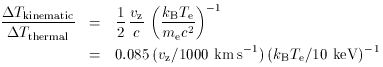(88)

which is small for the expected velocities of a few hundred km s-1 or less, and typical cluster temperatures of a few keV. However, the thermal and kinematic effects may be separated using their different spectra: indeed, in the Kompaneets approximation it is easy to show that the kinematic effect produces its maximum intensity change at the frequency at which the thermal effect is zero.

Thus observations near x = 3.83 (218 GHz) are sensitive mostly to the kinematic effect, but in interpreting such observations it is necessary to take careful account of the temperature-dependence of the shape of the thermal Sunyaev-Zel'dovich effect's spectrum, and of the frequency of the null of the thermal effect (Equation 60; Fig. 9), as emphasized by Rephaeli (1995a). The first strong limits on the peculiar velocities of clusters of galaxies derived using this technique are now becoming available (e.g., Holzapfel et al. 1997b; see Sec. 10.2).

Although this technique measures only the peculiar radial velocity of a cluster of galaxies, the other velocity components may be measured using the specific intensity changes caused by gravitational lensing (e.g., Birkinshaw & Gull 1983a, corrected by Gurvits & Mitrofanov 1986; Pyne & Birkinshaw 1993). These fractional intensity changes are small, of ordergrav (vxy / c), wheregrav is the gravitational lensing angle, and vxy is the velocity of the cluster across the observer's line of sight. (6) For typical cluster masses and sizes, the gravitational lensing angle is less than about 1 arcmin, so that (I / I)10-6 (vxy/1000 km s-1), whereas the kinematic Sunyaev-Zel'dovich effect may be an order of magnitude stronger. The possibility of measuring vz and vxy separately then depends on the different angular patterns of the effects on the sky: the transverse motion produces a characteristic dipole-like effect near the moving cluster, with an angular structure which indicates the direction of motion on the plane of the sky. Nevertheless, substantial improvements in techniques are going to be required to measure these other velocity terms in this way.

Clusters of galaxies produce further microwave background anisotropies through the same spacetime effect, if they are expanding or contracting (Nottale 1984; Pyne & Birkinshaw 1993). A contaminating Sunyaev-Zel'dovich effect must also appear at the same time if an expanding or collapsing cluster contains associated gas because of the anisotropy of inverse-Compton scattering (Molnar & Birkinshaw 1998b), but the sizes of these effects are too small to be detectable in the near future.

An interesting extension of this work would be to use the kinematic Sunyaev-Zel'dovich effect from a radio source to measure the speed of the radio-emitting plasma. Just as for a cluster of galaxies, the presence of a scattering medium which is moving relative to the CMBR will produce a kinematic Sunyaev-Zel'dovich effect which is proportional toe (vz / c), but whereas vz should be small for a cluster relative to the Hubble flow, the velocity of the radio-emitting plasma in a radio galaxy may be a substantial fraction of the speed of light, and a large kinematic Sunyaev-Zel'dovich effect will be seen if the optical depth of the radio-emitting plasma is sufficient. A complication here is that the location of the null of the non-thermal Sunyaev-Zel'dovich effect depends on the spectrum of the electrons, and that the cross-terms between the electron energy and the plasma velocity are no longer small. Thus the approximation of a cold plasma that was made in the derivation above, to calculate the spectrum of the kinematic Sunyaev-Zel'dovich effect, is no longer valid and a more complete calculation must be performed using proper angular averages over the (anisotropic) electron distribution function (see Molnar 1998).

6 This is a special case of a more general class of intensity-changing effects, often referred to as Rees-Sciama effects (after Rees & Sciama 1968), which arise when the evolution of spacetime near a cluster (or other massive object) differs from the evolution of the metric of the Universe as a whole (Pyne & Birkinshaw 1993). Back.